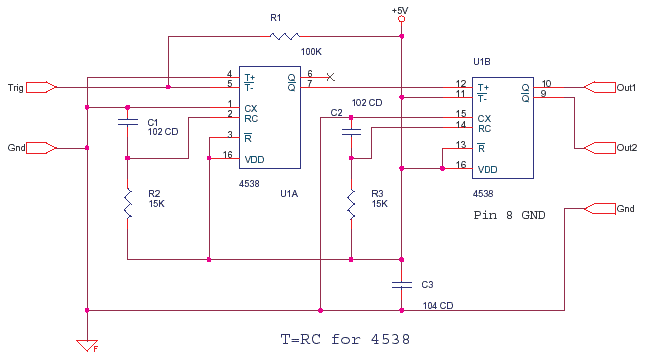Tuesday, July 07, 2015

Monostable Multivibrator CD4538

CD4538 is a dual Monostable Multivibrator. When you trigger the chip the output sends off one single pulse or one high-low event.

Mixed Circuits Analog with Digital

The T+ pin 4 of U1a is the positive edge trigger or raising edge trigger input, the T- pin 5 is falling edge or negative edge trigger input. Now see the image of the single pulse above which shows both the edges, If this is the input pulse at pin 5 then the falling edge turns the output pin 6 from low to high, this output remains high for time T = R2 * C1 and then goes low again, The output Q at pin 6 also looks like the image of pulse above.The Output pin 7 is the complementary state of pin 6, it is the reverse state or inverted form of pin 6 output.

Now why is a slope shown in the edges, this i have exaggerated a bit so that it can be explained. But then there is a slight slope due to gate input and output capacitance.

In fact if you had a wire or twisted track coming to the input and the R2C1 was in nano seconds, then you would see a ringing at the edges, a tiny peak or spike, which will have giga hertz frequency components, in fact a square way may be many sine waves put together, this you know from a spectrum analyzer.

Two Stage Sequential Timer

CD4538B can give an output with pulse width of 1uS and above. 74HC4538 gives 120nS to 60 Seconds pulses. The above circuit produces a pulse of width T = R3 * C2 after a delay of T = R2 * C1. Some Chips formula is T = 0.7 * R * C .